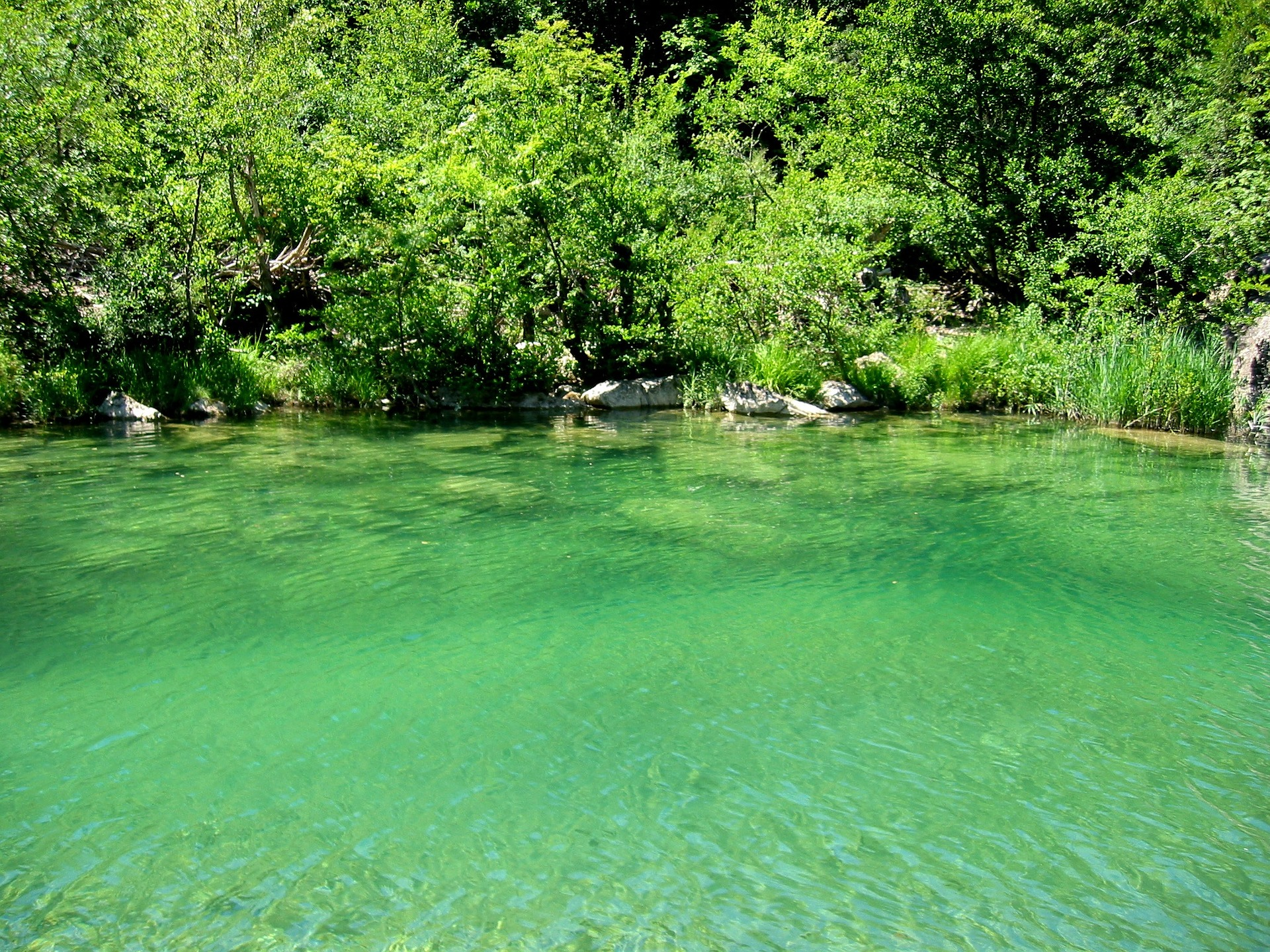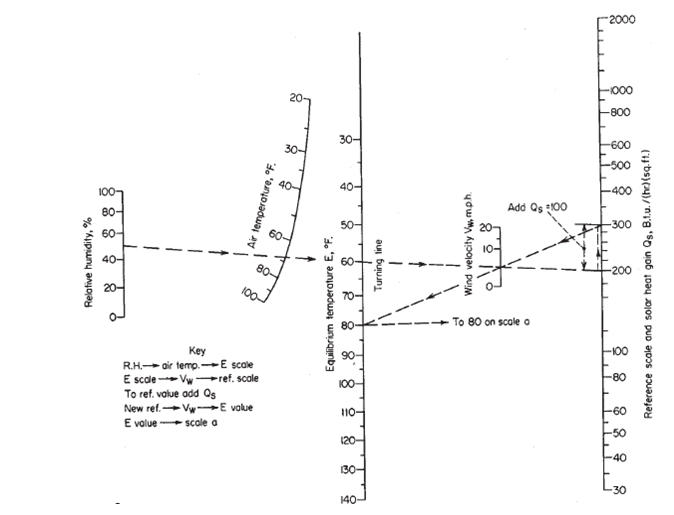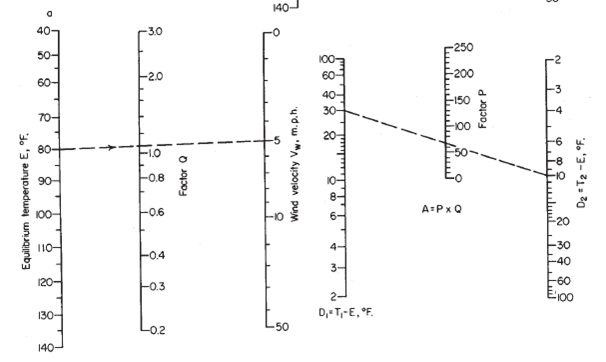# Cooling Pond Sizing

In this post, I want to share to you how to size cooling pond. Cooling pond is used to remove heat from water by means of air. Example of application of cooling pond is to remove heat from brine water in geothermal power plant facilities.

To design cooling pond, you need process data and environment data. Process data are flow rate of liquid to be cooled, initial temperature, final temperature, and density of liquid. Environent data are relative humidity, wind velocity, average air temperature, and solar heat gain. Here is example of cooling pond sizing.

We have these process data:

• Fluid type = brine water
• Brine mass flow = 1000 ton per hour
• Initial temperature = 45oC (113oF)
• Final temperature (after cooling) = 40oC (104oF)
• Brine density = 980 kg/m3

Environment data:

• Relative humidity = 80%
• Wind velocity = 7 mil per hour
• Average air temperature = 26oC (78.8oF)
• Solar heat gain = 65 Btu/(ft2.h). Solar heat gain is a function of lattitude and month.

Other data:

• Cooling pond is designed with additional 20% safety factor

Let’s start to estimate cooling pond size.

Step 1: Calculate volumetric flow rate of brine water.

Volumetric flow rate = brine mass flow x (1/density) x safety factor

= 1000 ton/h x 1000 kg/ton x (1/980 kg/m3) x (1+20%)

= 1224 m3/h (5388 gpm)

Step 2: Determine Equilibrium Temperature (Escale)

Equilibrium temperature or Escale is determined by using nomograph. It is a function of relative humidity and air temperature. At relative humidity of 80% and air temperature of 78.8oF, we get equilibrium temperature of 74oF.

Nomograph below is used to get data in Step 2 until Step 5.Step 3: Determine Reference Scale and Solar Heat Gain (Qscale)

Qscale is determined from Escale and wind velocity by using nomograph. With Escale 74oF and wind velocity of 7 mph, we get reference scale and solar heat gain (Qscale) of 300 Btu/(ft2.h).

Step 4: Add Qscale and Solar Heat Gain

Qscale = 300 Btu/(ft2.h)

Solar heat gain = 65 Btu/(ft2.h)

Qnew = Qscale + solar heat gain = 365 Btu/(ft2.h)

Step 5: Determine new equlibrium temperature (Evalue)

Evalue is determined by using nomograph. It is a function of Qnew and wind velocity. By using Qnew of 365 Btu/(ft2.h) and wind velocity of 7 mph, we get Evalue of 85oF.

Step 6: Determine Q factor

Q factor is determined by using the following nomograph. It is a function of equlibrium temperature (value in Step 5).

When Evalue is 85oF, we get Q factor of 0.9.Step 7: Determine D1 and D2

D1 = initial temperature of liquid – Evalue

= 113 – 85 = 28oF

D2 = final temperature of liquid – Evalue

= 104 – 85 = 19oF

Step 8: Determine P factor

P factor is determined by nomograph. It is a function of D1 and D2. When D1 is 28oF and D2 is 19oF, we get P factor of 20.

Step 9: Determine A

A = P factor x Q factor (Step 8 x Step 6)

= 20 x 0.9 = 18 ft2/gal/min

Step 10: Determine area of cooling pond

Area of cooling pond = A x volumetric flow rate (Step 9 x Step 1)

= 18 x 5388 = 96980 ft2 (9010 m2)

Step 11: Determine height of pond and volume of pond

If height of pond is 2.5 meter (8.2 ft), we get volume of pond is 20272 m3.

Last Step (Step 12): Check holding time of pond

Holding time of pond is = volume of pond/volumetric flow rate of brine

= 20272/1224 = 16.6 hours

I hope this step-by-step cooling pond sizing is easy to undestand and helpful.

Reference:

Perry’s Chemical Engineers’ Handbook.